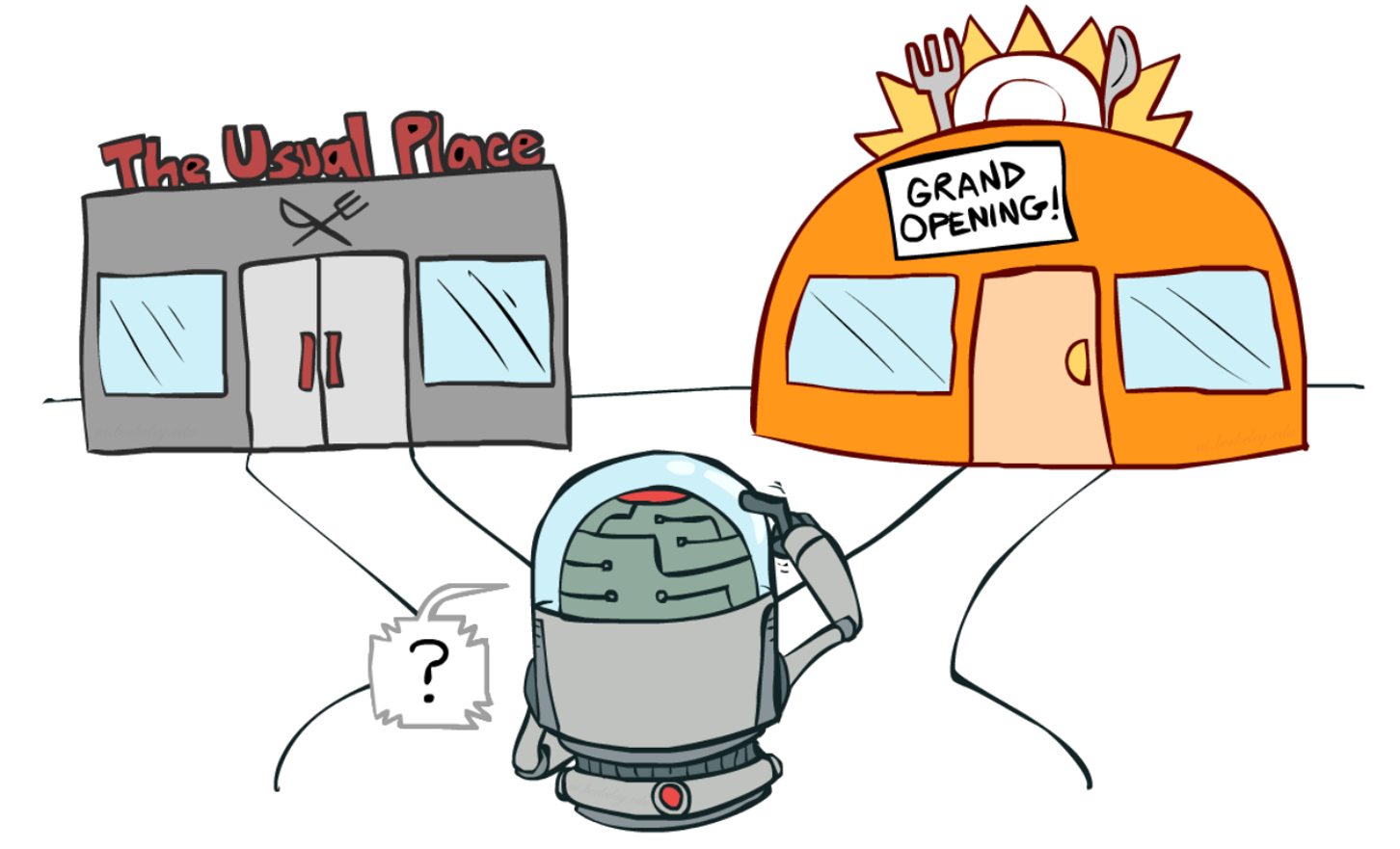# Thompson SamplingImagine having to make the following series of decisions. You have two drugs you can administer, drug 1, or drug 2. Initially you have no idea which drug is better. You want to know which drug is the most effective, but at the same time, there are costs to exploration — the stakes are high.

Here is an example:

Welcome to the drug simulator.
There are two drugs: 1 and 2.

Next patient. Which drug? (1 or 2): 1
Failure. Yikes!

Next patient. Which drug? (1 or 2): 2
Success. Patient lives!

Next patient. Which drug? (1 or 2): 2
Failure. Yikes!

Next patient. Which drug? (1 or 2): 1
Failure. Yikes!

Next patient. Which drug? (1 or 2): 1
Success. Patient lives!

Next patient. Which drug? (1 or 2): 1
Failure. Yikes!

Next patient. Which drug? (1 or 2): 2
Success. Patient lives!

Next patient. Which drug? (1 or 2): 2
Failure. Yikes!

Next patient. Which drug? (1 or 2): 

This problem is suprisingly complex. It sometimes goes by the name "the multi-armed bandit problem!" In fact, the perfect answer to this question can be exponentially hard to calculate. There are many approximate solutions and it is an active area of research.

One solution has risen to be a rather popular option: Thompson Sampling. It is easy to implement, elegant to understand, has provable garuntees , and in practice does very well .

### What You Know About The Choices

The first step in Thompson sampling is to express what you know (and what you do not know) about your choices. Let us revisit the example of the two drugs in the previous section. By the end we had tested drug 1 four times (with 1 success) and we had tested drug 2 four times (with 2 successes). A sophisticated way to represent our belief in the two hidden probabilites behind drug 1 and drug 2 is to use the Beta distribution. Let $X_1$ be the belief in the probability for drug 1 and let $X_2$ be the belief in the probability for drug 2. \begin{align*} X_1 \sim \Beta(a = 2, b = 4)\\ X_2 \sim \Beta(a = 3, b = 3) \end{align*}

Recall that in the Beta distribution with a uniform prior the first parameter, $a$, is number of observed successes + 1. The second parameter, $b$, is the number of observed fails + 1. It is helpful to look at these two distributions graphically:

If we had to guess, drug 2 is looking better, but there is still a lot of uncertainty, represented by the high variance in these beliefs. That is a helpful representation. But how can we use this information to make a good decision of the next drug.

### Making a Choice

It is hard to know what is the right choice! If you only had one more patient, then it is clear what you should do. You should calculate the probability that $X_2 > X_1$ and if that probability is over 0.5 then you should chose $a$. However, if you need to continually administer the pills then it is less clear what is the right choice. If you chose 1, you miss out on the chance to learn more about 2. What should we do? We need to balance this need for "exploring" and the need to take advantage of what we already know.

The simple idea behind Thompson Sampling is to randomly make your choice according to its probability of being optimal. In this case we should chose drug 1 with the probability that 1 is > 2. How do people do this in practice? They have a very simple formula. Take a random sample from each Beta distribution. Chose the option which has a larger value for its sample.

sample_a = sample_beta(2, 4)
sample_b = sample_beta(3, 3)
if sample_a > sample_b:
choose choice a
else:
choose choice b


What does it mean to take a sample? It means to chose a value according to the probability density (or probability mass) function. So in our example above, we might sample 0.4 for drug 1, and sample 0.35 for drug 2. In which case we would go with drug 1.

At the start Thompson Sampling "explores" quite a lot of time. As it gets more confident that one drug is better than another, it will start to chose that drug most of the time. Eventually it will converge to knowing which drug is best, and it will always chose that drug.